There are different types of equations that involve logs in some form or another and here I have broken down the types.

1. The the 1st tutorial shows how to solve equations where the unknown is a power.
2. In the 2nd tutorial I show you how to handle equations that contain log terms in the same base
3. In the 3rd tutorial I now look at the change of base identity where terms contain logs in different bases.
4. In the 4th tutorial I look at solving quadratic equations that contain exponential functions

Solving equations where the unknown is a power

In this tutorial you are shown how to solve equations where the unknown is a power.

Solve:

1.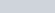2.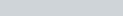Logs - How to solve equations where x is in the power : ExamSolutions Maths Revision - youtube Video

Solving equations that contain log terms

This next set of equations have logs in the equation. You may want to try these first before looking at the tutorial.

Solve:

1.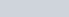2.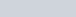3.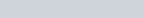4.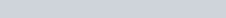Logs - How to Solve Equations that contain log terms : ExamSolutions Maths Revision - youtube Video

Solving equations that contain log terms in different bases using the change of base identity

In this tutorial you are shown how to solve equations that contain logarithms which are in different bases by using the change of base formula.

Solve:

1.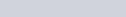Logs - Using the change of base identity in equations : ExamSolutions Maths Revision - youtube Video

Solving quadratic equations that contain exponential functions

In this tutorial you are shown how to solve quadratic equations in exponential functions. The solution often requires the use of logs.

Solve:

1.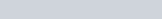2.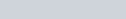Logs - Solving quadratic equations in a^x : ExamSolutions Maths Revision - youtube Video